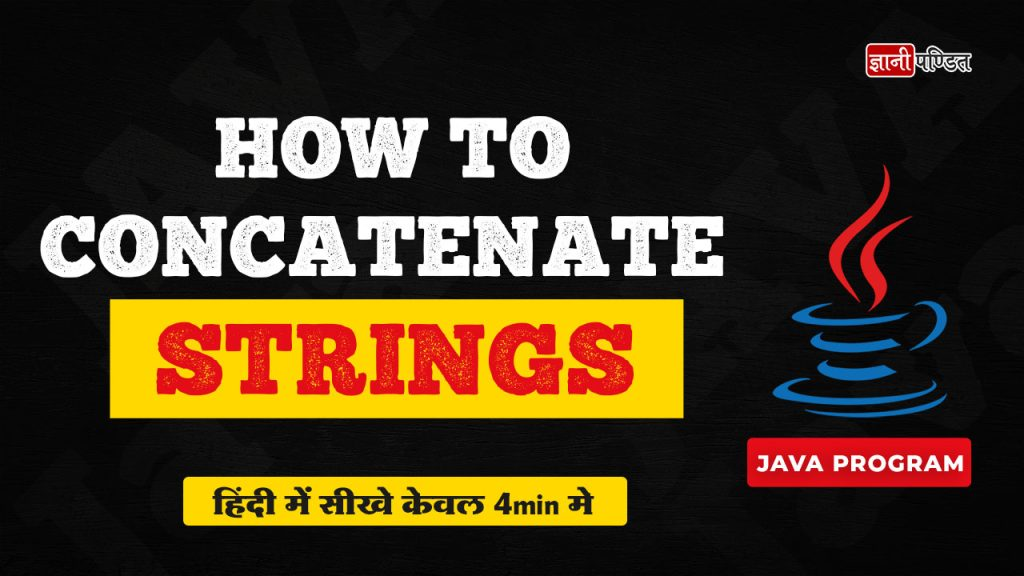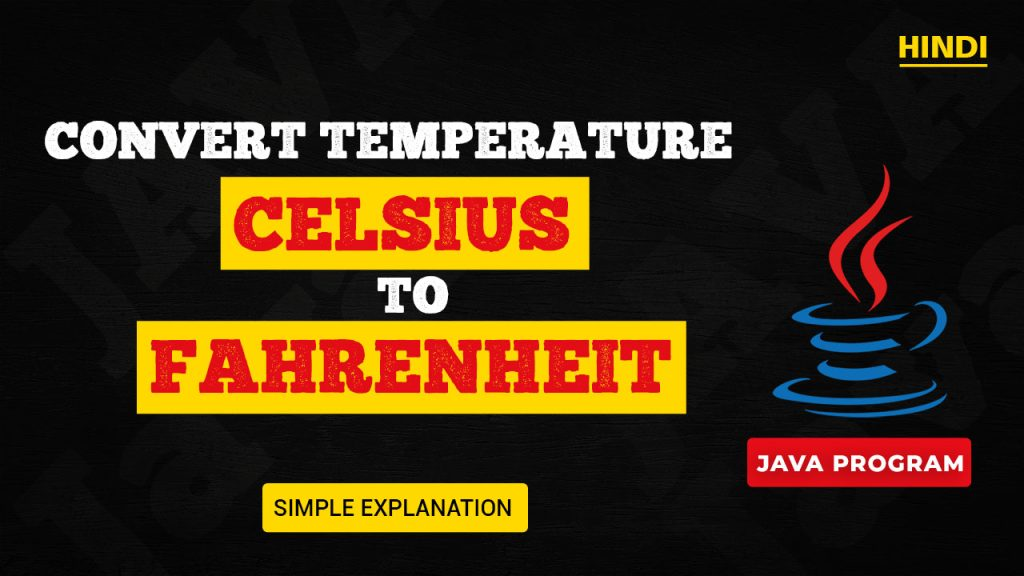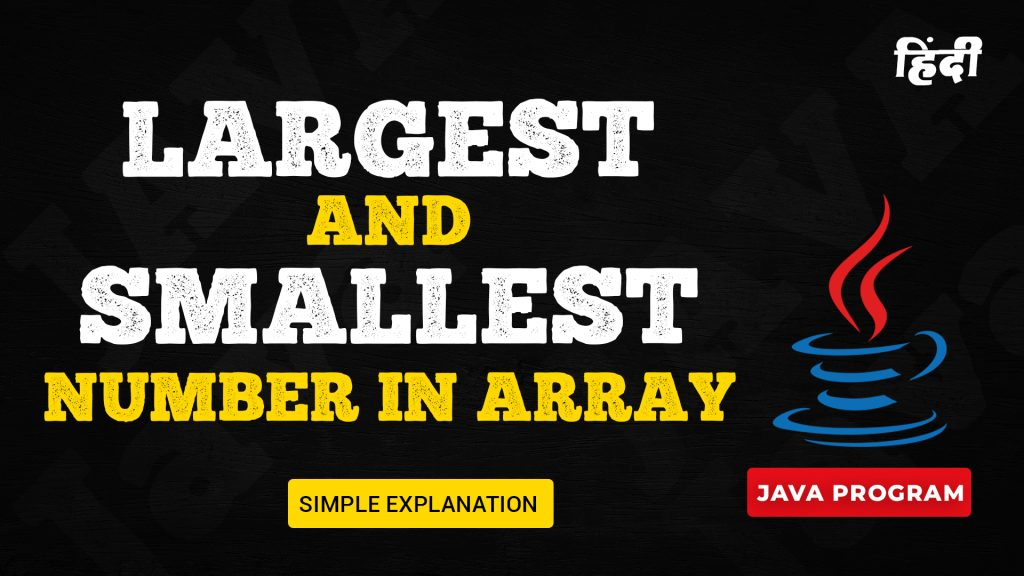# Java

## What is Java?

When it comes to programming, there are many different programming languages that are used for different purposes. Many people in this world, always have their eyes on the trend, and on the most popular and most widely used programming languages in this world. One such very widely used, and very popular programming language is Java. …## How to Concatenate Strings in Java

In this video, we are going to learn about how can we concatenate strings in Java. At times, In our Java programs, we might require to concatenate strings, and in such situations, we can concatenate the strings by doing some simple stuff. We can make use of the + operator with the strings, to concatenate …## Convert Celsius to Fahrenheit Program in Java

Here, we are performing a simple Java program, to convert the temperature from degree Celsius to degree Fahrenheit. To convert the temperature from degree Celsius to degree Fahrenheit, we just need to make use of a simple conversion formula. You can also try to perform this simple program, and many such others simple conversion programs, …

## Java Program to Find Largest Number in an Array

In this article, we are going to understand and perform a simple task in Java, in which, we are given an array, and we need to find the largest number in the array. We are required to write a Java program for the same. So, we will first understand the logic behind the program, and …

## How to find the first and last digit of a number in Java

In this article, we are going to understand and perform a simple Java program, in which, we are going to find the first and last digits of a given number. For example, the number we have is 1234, so the first and last digits that we need to have here are 1 and 4 respectively. …## Largest and Smallest Number in Array Java Program

In this article, we are going to understand and write a simple Java program, to find the largest and smallest numbers in the array. We would first understand how are we going to achieve this task, and then we are going to perform a Java program for the same. As a prerequisite for doing this …

## Difference between print and println in Java

When it comes to printing some output in Java, we have got some methods, like print, println, etc. In this article, we are going to discuss some points of differences between print and println methods. We would list the points of difference, and also, we would also try to have some practical examples to understand …## Java Program to Convert Celsius to Fahrenheit

Celsius to Fahrenheit Conversion In this article, we are going to understand and implement a simple Java program, to convert the temperature from degrees Celsius to Degrees Fahrenheit. This program is going to be a simple formula-based program, in which, we are going to get the temperature in degree Celsius from the user, and using …